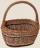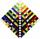# AVG of INT

What is the average of the integers from 9 throuht 52 inclusive?

Result

x =  30.5

#### Solution:Leave us a comment of example and its solution (i.e. if it is still somewhat unclear...):Be the first to comment!#### To solve this example are needed these knowledge from mathematics:

Looking for help with calculating arithmetic mean? Looking for a statistical calculator?

## Next similar examples:

1. Sum of membersWhat is the sum of the first two members of the aritmetic progression if d = -4.3 and a3 = 7.5?
2. AverageThe arithmetic mean of the two numbers is 71.7. One number is 5. Calculate the second number.
3. TreesA certain species of tree grows an average of 0.5 cm per week. Write an equation for the sequence that represents the weekly height of this tree in centimeters if the measurements begin when the tree is 200 centimeters tall.
4. Median and modusRadka made 50 throws with a dice. The table saw fit individual dice's wall frequency: Wall Number: 1 2 3 4 5 6 frequency: 8 7 5 11 6 13 Calculate the modus and median of the wall numbers that Radka fell.
5. 75th percentile (quartille Q3)Find 75th percentile for 30,42,42,46,46,46,50,50,54
6. Sequence 2Write the first 5 members of an arithmetic sequence a11=-14, d=-1Determine how many apples are in baskets when in the first basket are 4 apples, and in any other is 29 apples more than the previous, and we have eight baskets.
8. Nineteenth memberFind the nineteenth member of the arithmetic sequence: a1=33 d=5 find a19
9. Sequence 3Write the first 5 members of an arithmetic sequence: a4=-35, a11=-105.Combine like terms 4c+c-7c
11. SequenceWrite the first 6 members of these sequence: a1 = 5 a2 = 7 an+2 = an+1 +2 an
12. SeriesYour task is express the sum of the following arithmetic series for n = 14: S(n) = 11 + 13 + 15 + 17 + ... + 2n+9 + 2n+11
13. SeatsSeats in the sport hall are organized so that each subsequent row has five more seats. First has 10 seats. How many seats are: a) in the eighth row b) in the eighteenth row
14. AP - simpleDetermine the first nine elements of sequence if a10 = -1 and d = 4
15. SequenceBetween numbers 1 and 53 insert n members of the arithmetic sequence that its sum is 702.
16. SequenceWrite the first 7 members of an arithmetic sequence: a1=-3, d=6.
17. 6 termsFind the first six terms of the sequence. a1 = 7, an = an-1 + 6